Highest Daily Dewpoint Climatology for Paducah, KY (September 1949 - June 2015)

Min = Minimum daily high dewpoint temperature recorded during that time period

10th Percentile = 10% chance that the highest daily dewpoint will fall below this threshold for that time period. Anything below the 10th percentile is considered unseasonably low. Another way to view it is that 90% of the time the daily high dewpoint will be higher than this specified threshold.

Median = Basically the average daily high dewpoint; there is an equal probability of dewpoints falling above or below this value.

90th Percentile = 10% chance that the highest daily dewpoint will fall above this threshold for that time period. Anything above the 90th percentile is considered unseasonably high. Another way to view it is that 90% of the time the daily high dewpoint will be lower than this specified threshold.

Max = Highest dewpoint temperature recorded during that time period

Dewpoints between the 25th and 75th percentile are the most common for that time period.

*NOTE: This data is only for the highest dewpoint recorded on a given day and does not take into account the lowest or average dewpoint for the whole day. The highest dewpoint is a good measure of the moisture content of the air. In the cool season, there is also an enhanced potential at severe weather occurring if the dewpoint reaches into the upper threshold (generally 95th percentile and above) for a given month.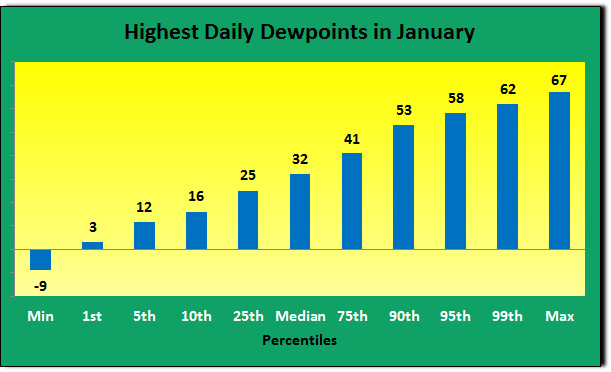January # of Days % of Days Dewpoints >=65 2 0.1% Dewpoints >=60 60 3.3% Dewpoints >=50 244 13.6% Dewpoints >=40 507 28.3% Dewpoints >=30 1122 62.5% Total Count: 1794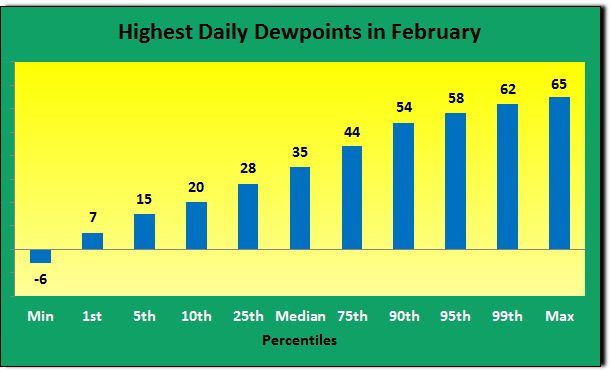February # of Days % of Days Dewpoints >=65 1 0.1% Dewpoints >=60 46 2.8% Dewpoints >=50 272 16.6% Dewpoints >=40 568 34.7% Dewpoints >=30 1172 71.6% Total Count: 1637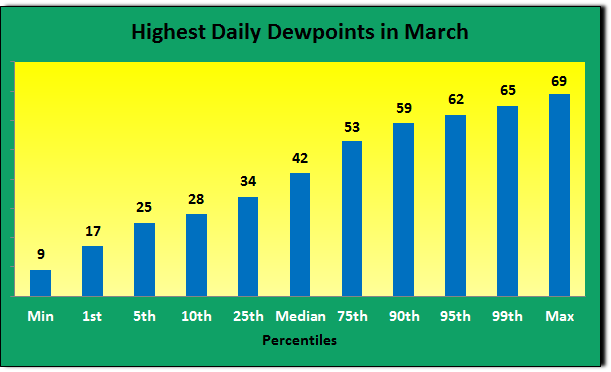March # of Days % of Days Dewpoints >=65 19 1.1% Dewpoints >=60 161 9.0% Dewpoints >=50 556 30.9% Dewpoints >=40 1056 58.7% Dewpoints >=30 1562 86.9% Total Count: 1798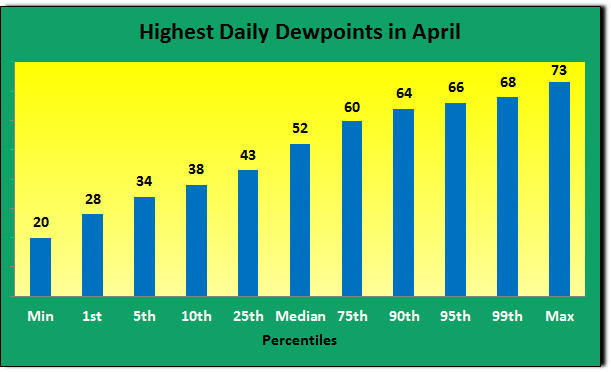April # of Days % of Days Dewpoints >=70 9 0.5% Dewpoints >=65 158 9.1% Dewpoints >=60 486 28.0% Dewpoints >=50 984 56.6% Dewpoints >=40 1487 85.6% Dewpoints >=30 1713 98.6% Total Count: 1738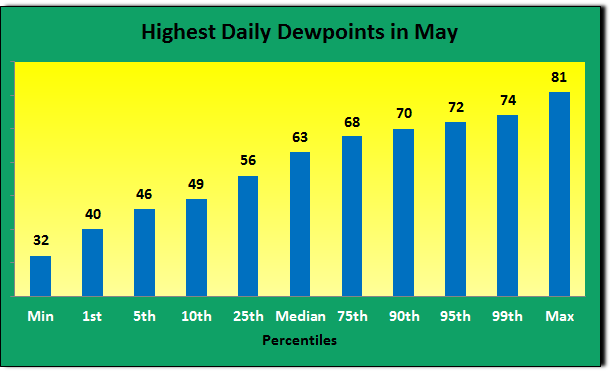May # of Days % of Days Dewpoints >=80 1 0.1% Dewpoints >=75 11 0.6% Dewpoints >=70 252 14.0% Dewpoints >=65 751 41.8% Dewpoints >=60 1118 62.2% Dewpoints >=50 1609 89.6% Total Count: 1796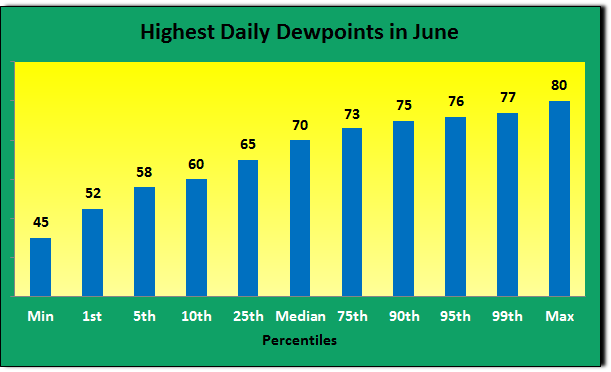June # of Days % of Days Dewpoints >=80 1 0.1% Dewpoints >=75 202 11.6% Dewpoints >=70 930 53.5% Dewpoints >=65 1340 77.1% Dewpoints >=60 1594 91.7% Total Count: 1738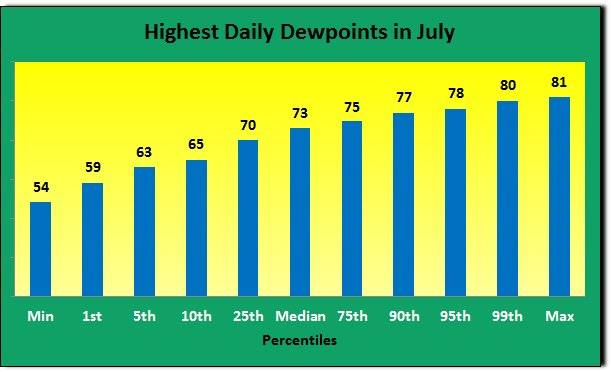July # of Days % of Days Dewpoints >=80 25 1.4% Dewpoints >=75 609 34.4% Dewpoints >=70 1359 76.9% Dewpoints >=65 1617 91.5% Dewpoints >=60 1741 98.5% Total Count: 1768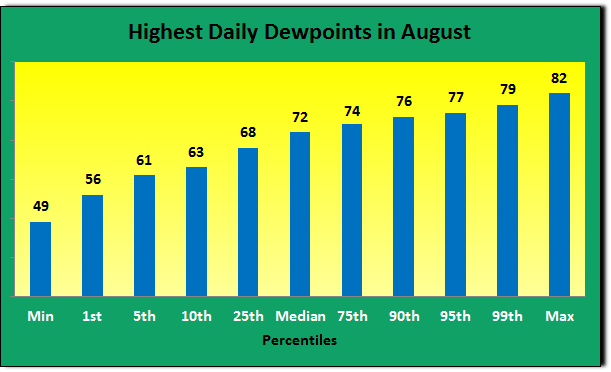August # of Days % of Days Dewpoints >=80 16 0.9% Dewpoints >=75 413 23.4% Dewpoints >=70 1171 66.3% Dewpoints >=65 1534 86.8% Dewpoints >=60 1710 96.8% Total Count: 1767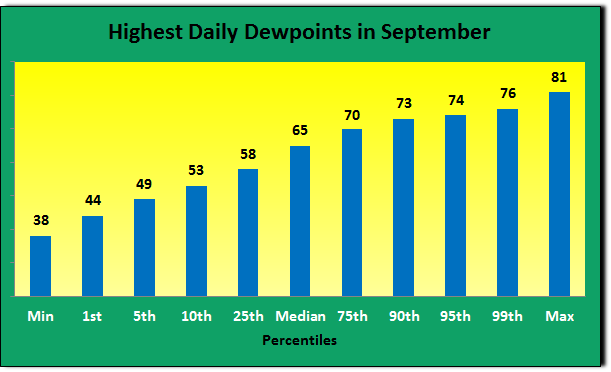September # of Days % of Days Dewpoints >=80 2 0.1% Dewpoints >=75 60 3.4% Dewpoints >=70 498 28.6% Dewpoints >=65 934 53.7% Dewpoints >=60 1234 70.9% Dewpoints >=50 1646 94.6% Total Count: 1740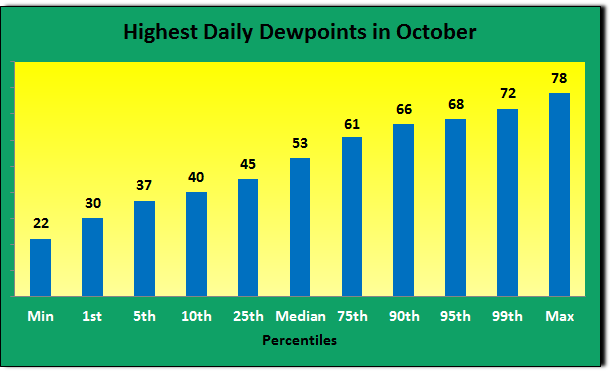October # of Days % of Days Dewpoints >=75 2 0.1% Dewpoints >=70 60 3.3% Dewpoints >=65 258 14.4% Dewpoints >=60 535 29.8% Dewpoints >=50 1127 62.8% Dewpoints >=40 1627 90.6% Total Count: 1795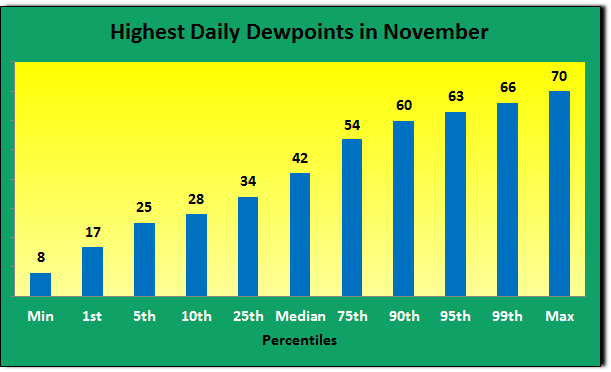November # of Days % of Days Dewpoints >=70 1 0.1% Dewpoints >=65 49 2.8% Dewpoints >=60 207 11.9% Dewpoints >=50 581 33.4% Dewpoints >=40 1011 58.2% Dewpoints >=30 1516 87.2% Total Count: 1738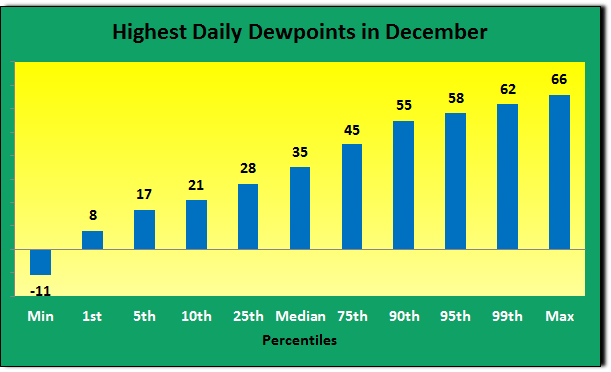December # of Days % of Days Dewpoints >=65 7 0.4% Dewpoints >=60 60 3.3% Dewpoints >=50 321 17.8% Dewpoints >=40 666 37.0% Dewpoints >=30 1251 69.5% Total Count: 1799